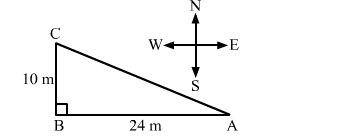# A man goes 24 m due west and them 10 m due north. How far is he from the starting point?Question:

A man goes 24 m due west and them 10 m due north. How far is he from the starting point?
(a) 34 m
(b) 17 m
(c) 26 m
(d) 28 m

Solution:

(c) 26 mSuppose, the man starts from point A and goes 24 m due west to point B. From here, he goes 10 m due north and stops at C.
In right triangle ABC, we have:
AB = 24 m, BC = 10 m
Applying Pythagoras theorem, we get:

$A C^{2}=A B^{2}+B C^{2}=24^{2}+10^{2}$

$A C^{2}=576+100=676$

$A C=\sqrt{676}=26$